Download the eSaral app and start learning from Kota's top IITians and doctors.

JEE Advanced Previous Year Questions of Math with Solutions are available at eSaral. Practicing JEE Advanced Previous Year Papers Questions of mathematics will help the JEE aspirants in realizing the question pattern as well as help in analyzing weak & strong areas. eSaral helps the students in clearing and understanding each topic in a better way. eSaral also provides complete chapter-wise notes of Class 11th and 12th both for all subjects. Besides this, eSaral also offers NCERT Solutions, Previous year questions for JEE Main and Advance, Practice questions, Test Series for JEE Main, JEE Advanced and NEET, Important questions of Physics, Chemistry, Math, and Biology and many more. Download eSaral app for free study material and video tutorials.     Simulator   Previous Years JEE Advanced Questions
Q. The smallest value of $\mathrm{k},$ for which both the roots of the equation, $\mathrm{x}^{2}-8 \mathrm{kx}+16\left(\mathrm{k}^{2}-\mathrm{k}+1\right)=0$ are real, distinct and have values at least $4,$ is [JEE 2009, 4 (–1)]
Ans. 2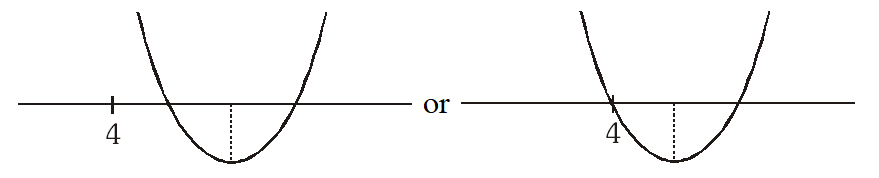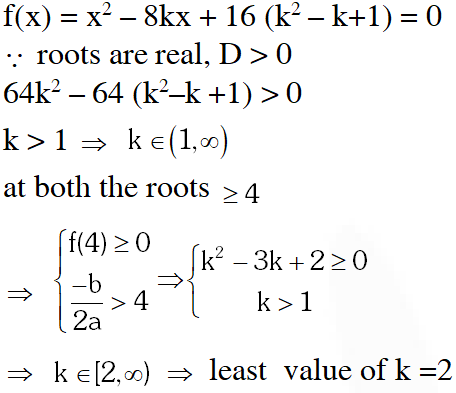Q. Let p and q be real numbers such that $p \neq 0, p^{3} \neq q$ and $p^{3} \neq-q .$ If $\alpha$ and $\beta$ are nonzero complex numbers satisfying $\alpha+\beta=-p$ and $\alpha^{3}+\beta^{3}=q$, then a quadratic equation having $\frac{\alpha}{\beta}$ and $\frac{\beta}{\alpha}$ as its roots is (A) $\left(p^{3}+q\right) x^{2}-\left(p^{3}+2 q\right) x+\left(p^{3}+q\right)=0$ (B) $\left(\mathrm{p}^{3}+\mathrm{q}\right) \mathrm{x}^{2}-\left(\mathrm{p}^{3}-2 \mathrm{q}\right) \mathrm{x}+\left(\mathrm{p}^{3}+\mathrm{q}\right)=0$ (C) $\left(\mathrm{p}^{3}-\mathrm{q}\right) \mathrm{x}^{2}-\left(5 \mathrm{p}^{3}-2 \mathrm{q}\right) \mathrm{x}+\left(\mathrm{p}^{3}-\mathrm{q}\right)=0$ (D) $\left(p^{3}-q\right) x^{2}-\left(5 p^{3}+2 q\right) x+\left(p^{3}-q\right)=0$ [JEE 2010, 3]
Ans. (B)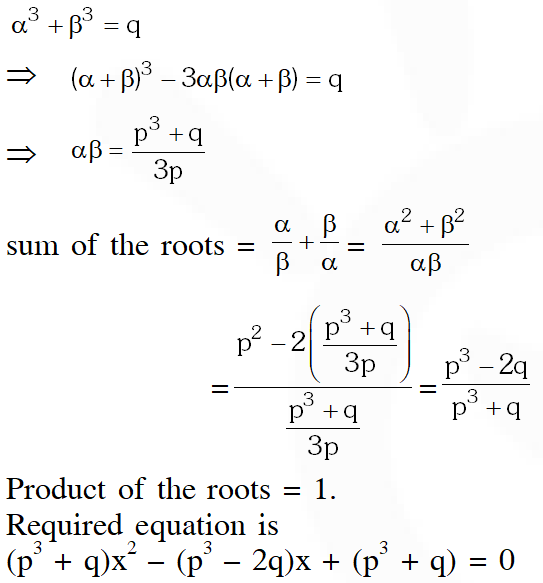Q. Let $\alpha$ and $\beta$ be the roots of $\mathrm{x}^{2}-6 \mathrm{x}-2=0,$ with $\alpha>\beta .$ If $\mathrm{a}_{\mathrm{n}}=\alpha^{\mathrm{n}}-\beta^{\mathrm{n}}$ for $\mathrm{n} \geq 1,$ then the value of $\frac{\mathrm{a}_{10}-2 \mathrm{a}_{8}}{2 \mathrm{a}_{9}}$ is (A) 1              (B) 2             (C) 3             (D) 4 [JEE 2011]
Ans. (C)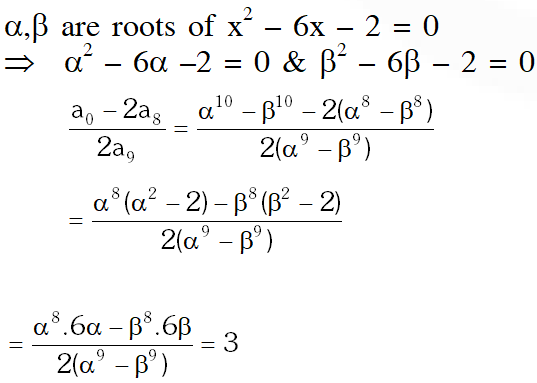Q. A value of b for which the equations $\mathrm{x}^{2}+\mathrm{bx}-1=0$ $\mathrm{x}^{2}+\mathrm{x}+\mathrm{b}=0$ have one root in common is – (A) $-\sqrt{2}$ (B) $-i \sqrt{3}$ (C) $\mathrm{i} \sqrt{5}$ (D) $\sqrt{2}$ [JEE 2011]
Ans. (B)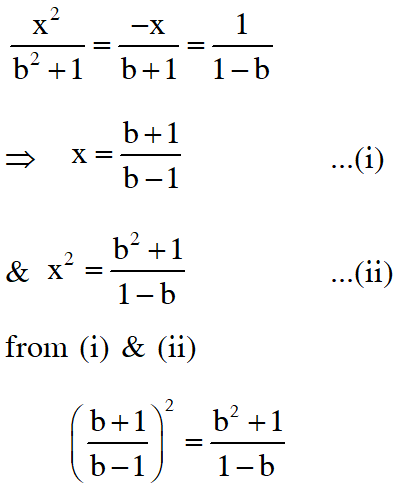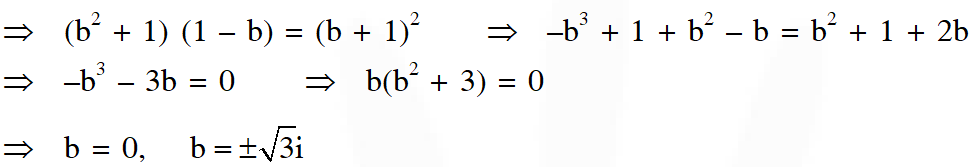Q. Let S be the set of all non-zero numbers a such that the quadratic equation $\alpha \mathrm{x}^{2}$ – x + a = 0 has two distinct real roots $\mathrm{x}_{1}$ and $\mathrm{x}_{2}$ satisfying the inequality $\left|x_{1}-x_{2}\right|<1$. Which of the following intervals is(are) a subset(s) of S ? (A) $\left(-\frac{1}{2},-\frac{1}{\sqrt{5}}\right)$ (B) $\left(-\frac{1}{\sqrt{5}}, 0\right)$ (C) $\left(0, \frac{1}{\sqrt{5}}\right)$ (D) $\left(\frac{1}{\sqrt{5}}, \frac{1}{2}\right)$ [JEE 2015, 4M, –0M]
Ans. (A,D)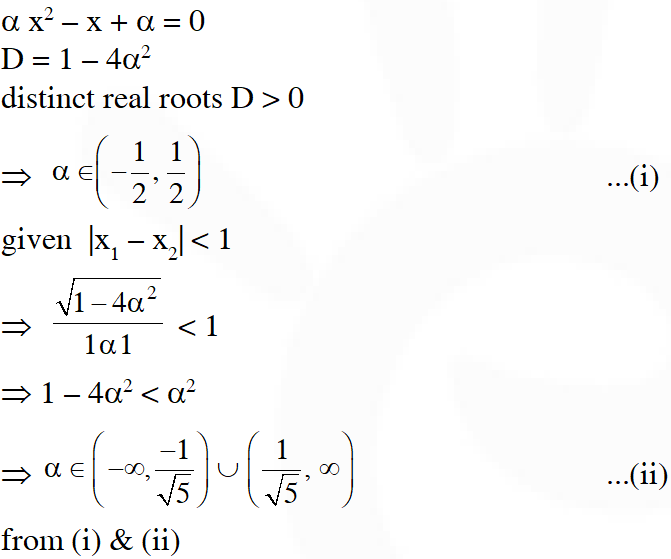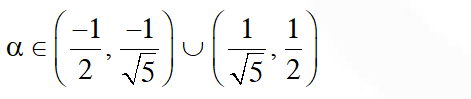Q. Let $-\frac{\pi}{6}<\theta<-\frac{\pi}{12}$. Suppose $\alpha_{1}$ and $\beta_{1}$ are the roots of the equation $\mathrm{x}^{2}-2 \mathrm{xsec} \theta+1=0$ and $\alpha_{2}$ and $\beta_{2}$ are the roots of the equation $\mathrm{x}^{2}+2 \mathrm{xtan} \theta-1=0$. If $\alpha_{1}>\beta_{1}$ and $\alpha_{2}>\beta_{2},$ then $\alpha_{1}+\beta_{2}$ equals (A) $2(\sec \theta-\tan \theta)$ (B) $2 \sec \theta$ (C) $-2 \tan \theta$ (D) 0 [JEE(Advanced)-2016, 3(–1)]
Ans. (C)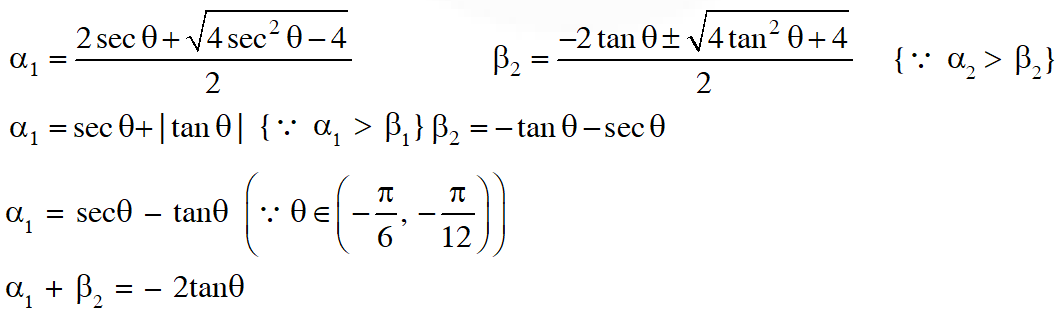PARAGRAPH 2 Let p,q be integers and let $\alpha, \beta$ be the roots of the equation, $x^{2}-x-1=0,$ where $\alpha \neq \beta .$ For $n=0,1,2, \ldots . .,$ let $a_{n}=p \alpha^{n}+q \beta^{n}$. FACT : If a and b are rational numbers and $a+b \sqrt{5}=0,$ then $a=0=b$.
Q. If $a_{4}=28,$ then $p+2 q=$ (A) 14 (B) 7 (C) 12 (D) 21 [JEE(Advanced 2017, 3(–1)]
Ans. (C)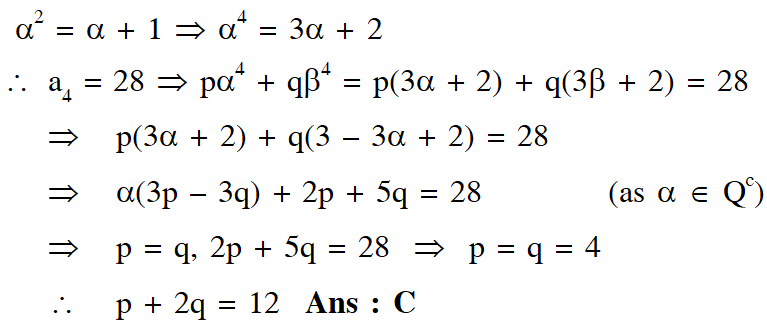Q. $\mathrm{a}_{12}=$ (A) $2 \mathrm{a}_{11}+\mathrm{a}_{10}$ (B) $a_{11}-a_{10}$ (C) $\mathrm{a}_{11}+\mathrm{a}_{10}$ (D) $a_{11}+2 a_{10}$ [JEE(Advanced 2017, 3(–1)]
Ans. (C)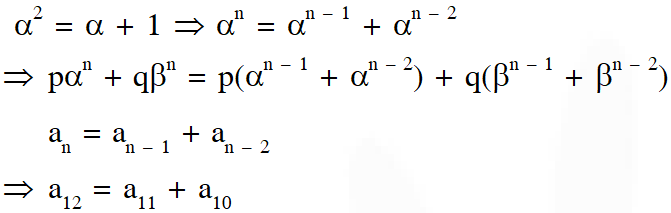BGMI LOVER
Feb. 5, 2023, 11:21 p.m.
Great questions I will suggest everyone to join E saral
Es
May 6, 2022, 6:49 p.m.
Tyyfyfdtyutrgreet Fgdgdgrwetrysr
Balamurugan
July 25, 2021, 10:59 a.m.
Sir one doubt if e = q/eo = [E] = rsinΦdΦ = q/eo = 4πr*2[e] = r = 14631m = 1/4π(14631)*8.85×10*-9f/m = 42.00479 Is this meaning of life sir
Vinit jangir
June 12, 2021, 2:29 p.m.
Sir my me is Vinit and I am taking lecture of Saransh sir and now I cracked jee advanced 2020 with self study and e saral YouTube videos got AIR 47
Jaat
May 19, 2023, 3:02 p.m.
Chutita kaat rha h
hi
May 12, 2021, 9:25 p.m.
awesome question
Keshav
May 11, 2021, 9:31 p.m.
Last one paragraph is best. But i am disappointed because there was only 7 or 8 question 😖. But thanks for that
Nitin jangid
April 26, 2021, 5:21 p.m.
Good question🙋🙋🙋
Divyansh Shrivastava
March 9, 2021, 5:03 p.m.
Thank You very much for these questions
GB
Oct. 19, 2020, 9:30 a.m.
Awesome questions
Yashwanth
Oct. 15, 2020, 10:19 p.m.
Really helpful and the solutions are really good and very concise.
Anish
Oct. 14, 2020, 10:47 a.m.
Really good
Vikas
Sept. 19, 2020, 4:58 p.m.